Question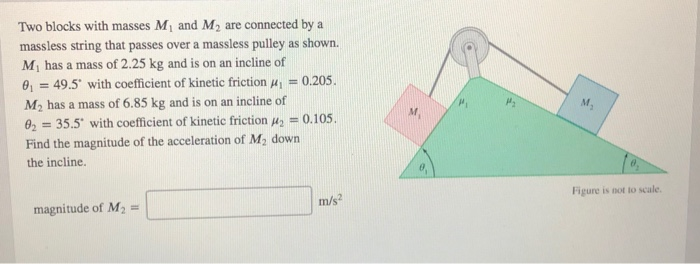We need at least 10 more requests to produce the answer.

0 / 10 have requested this problem solution

The more requests, the faster the answer.

All students who have requested the answer will be notified once they are available.

#### Earn Coins

Coins can be redeemed for fabulous gifts.

Similar Homework Help Questions
• ### Two blocks with masses M1 and M2 are connected by a massless string that passes over a massless pulley as shown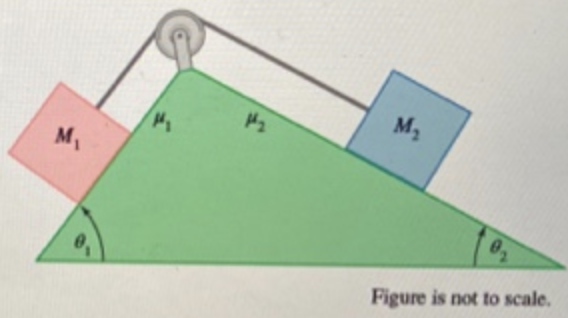Two blocks with masses M1 and M2 are connected by a massless string that passes over a massless pulley as shown. M1 has a mass of 2.25 kg and is on an incline of θ1=43.5° with coefficient of kinetic friction μ1=0.205 . M2 has a mass of 6.15 kg and is on an incline of θ2=35.5° with coefficient of kinetic friction μ2=0.105. The two-block system is in motion with the block of mass M2 sliding down the ramp.Find the magnitude...

• ### Two blocks with masses M1 and M2 are connected by a massless string that passes over...

Two blocks with masses M1 and M2 are connected by a massless string that passes over a massless pulley as shown. M1 has a mass of 2.25 kg and is on an incline of 41.5° with coefficient of kinetic friction μ1 = 0.205. M2 has a mass of 6.25 kg and is on an incline of 31.5° with coefficient of kinetic friction μ2 = 0.105. Find the magnitude of the acceleration of M2 down the incline.

• ### w Expand Question 13 of 20 Two blocks with masses M and M2 are connected by...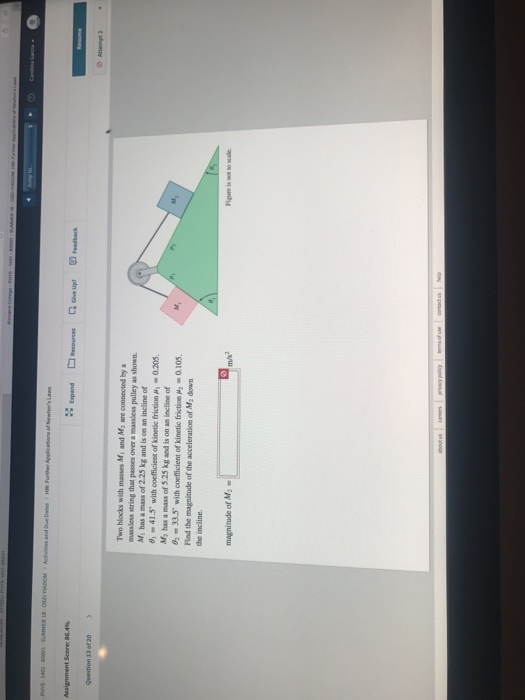w Expand Question 13 of 20 Two blocks with masses M and M2 are connected by massless string that passes over a massless pulley as shown Mi has a mass of 2.25 kg and is on an incline of o,-41.5 with coefficient of kinetic friction M-0205. M2 has a mass of 525 kg and is on an incline of 33.5 with coefficient of kinetic friction M 0.105 Find the magnitude of the acceleration of M2 down the incline. magnitude of...

• ### Two blocks of masses M1 and M2 are connected by a massless string that passes over...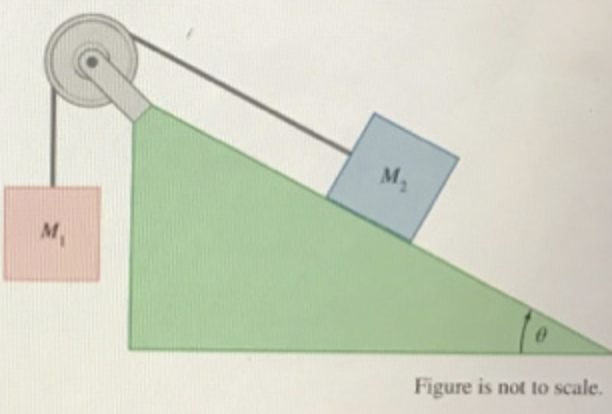Two blocks of masses M1 and M2 are connected by a massless string that passes over a massless pulley as shown in the figure. M2. which has a mass of 13.5 kg, rests on a long ramp of angle θ=15.5°. Friction can be ignored in this problem. Find the value of the mass Mi for which the two blocks are in equilibrium (i.e., not accelerating).

• ### Two blocks of masses M and M2 are connected by a massless string that passes over...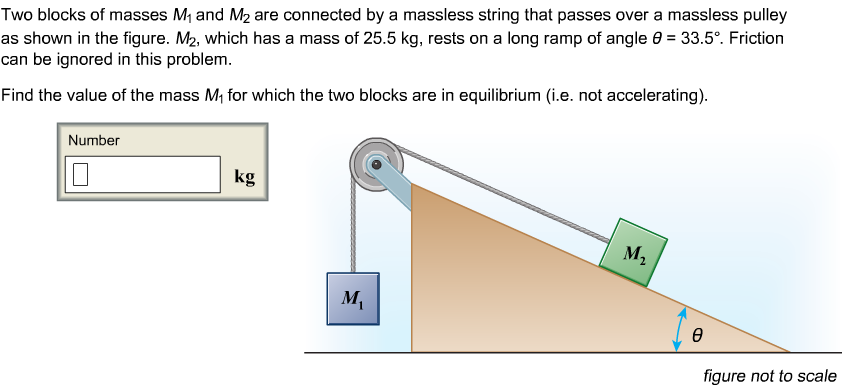Two blocks of masses M and M2 are connected by a massless string that passes over a massless pulley as shown in the figure. M2, which has a mass of 25.5 kg, rests on a long ramp of angle θ-33.5. Friction can be ignored in this problem Find the value of the mass M1 for which the two blocks are in equilibrium (i.e. not accelerating) Number kg figure not to scale

• ### Two blocks of masses Mi and M2 are connected by a massless string that passes over...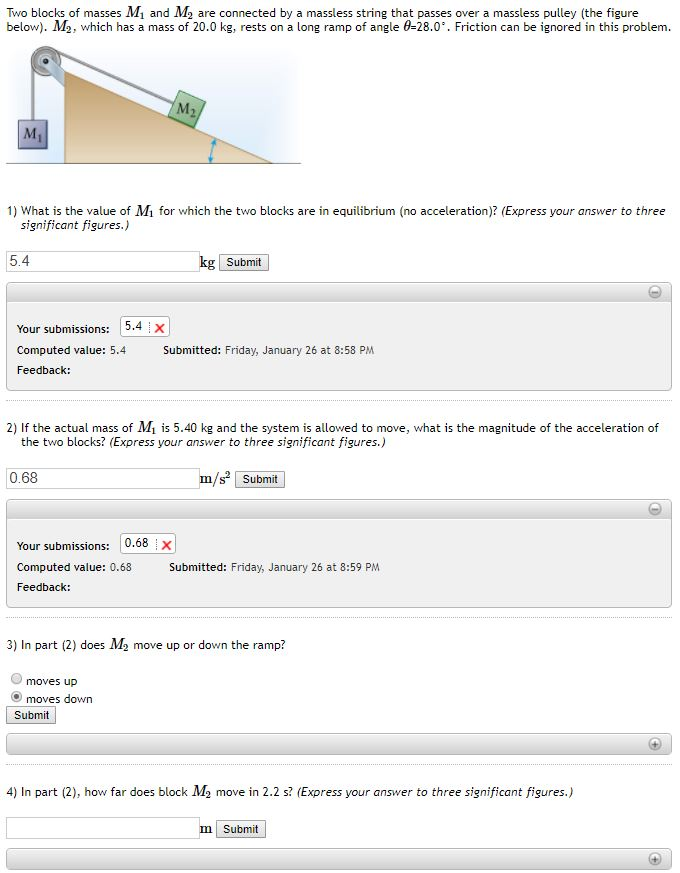Two blocks of masses Mi and M2 are connected by a massless string that passes over a massless pulley (the figure below). M2, which has a mass of 20.0 kg, rests on a long ramp of angle 428.0°. Friction can be ignored in this problem. 1) What is the value of Mi for which the two blocks are in equilibrium (no acceleration)? (Express your answer to three significant figures.) 5.4 Submit Your submissions: 15.4 Computed value: 5.4 Feedback: Submitted: Friday,...

• ### Two blocks with masses m1 and m2 are connected by a massless string over a frictionless...

Two blocks with masses m1 and m2 are connected by a massless string over a frictionless pulley. Block 1 sits on a frictionless horizontal surface and block 2 sits on a plane inclined at an angle θ above the horizontal. The coefficient of friction between block 2 and the incline is µk. The pulley, which is a uniform disk, has a mass mp and a radius R. When you release the blocks, both blocks slide without the string slipping on...

• ### Problem 2: Consider two blocks of masses mi and m2 connected by a massless cable. The...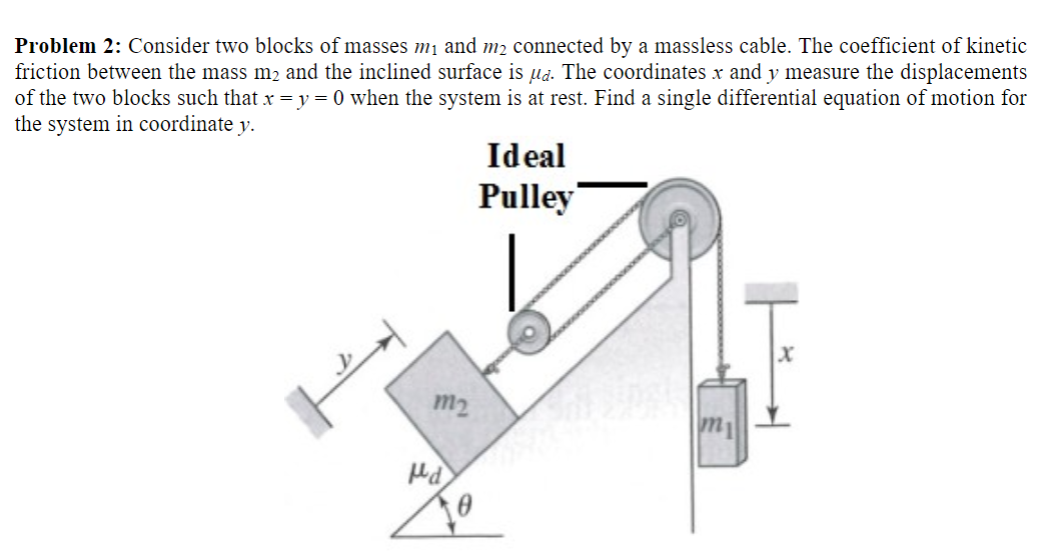Problem 2: Consider two blocks of masses mi and m2 connected by a massless cable. The coefficient of kinetic friction between the mass m2 and the inclined surface is ud. The coordinates x and y measure the displacements of the two blocks such that x=y=0 when the system is at rest. Find a single differential equation of motion for the system in coordinate y. Ideal Pulley m2 d

• ### Two blocks with mass m1 = 6.8 kg and m2 = 7.3 kg are connected by a massless string over a frictionless and massless pulley

Two blocks with mass m1 = 6.8 kg and m2 = 7.3 kg are connected by a massless string over a frictionless and massless pulley. The angle of the incline is equal to 32.5°. The kinetic coefficient of friction between m1 and the incline is 0.21. (a)What is the minimum value of the static friction coefficient that will prevent m1 from starting to move if it is at rest. (b)Find the magnitude of the acceleration of the system if m1...

• ### Two blocks m1=8.1 kg and mass m2 are connected by a massless cord over a massless...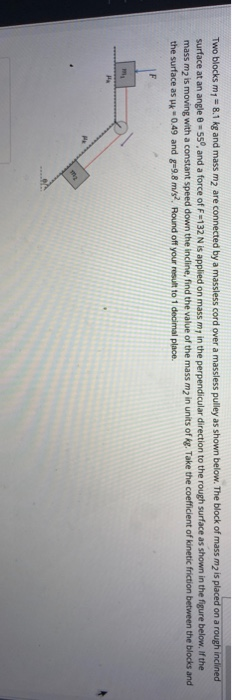Two blocks m1=8.1 kg and mass m2 are connected by a massless cord over a massless pulley as shown below. the block of mass m2 is placed on a rough inclined surface at an angle (theta = 55) Two blocks my = 8.1 kg and mass m2 are connected by a massless cord over a massless pulley as shown below. The block of mass mz is placed on a rough inclined surface at an angle = 55°, and a force...